# Power System Stability

## Recent Trends in Power System Stability

Recent Trends in Power System Stability: Recent Trends in Power System Stability in design of large alternators tend towards lower short circuit ratio (SCR = 1/Xd), which is achieved by reducing machine air gap with consequent savings in machine mmf, size, weight and cost. Reduction in the size of rotor reduces inertia constant, lowering thereby …

## Factors Affecting Transient Stability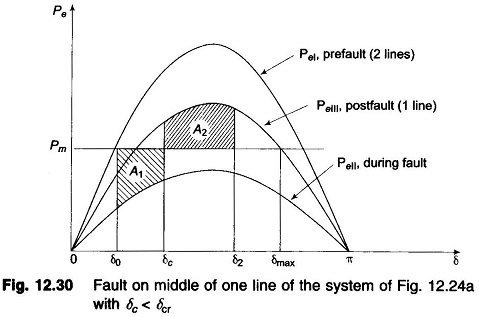Factors Affecting Transient Stability: Factors Affecting Transient Stability – The two-machine system can be equivalently reduced to a single machine connected to infinite bus bar. The qualitative conclusions regarding system stability drawn from a two-machine or an equivalent one-machine infinite bus system can be easily extended to a multimachine system. In the last article we …

## Numerical Solution of Swing Equation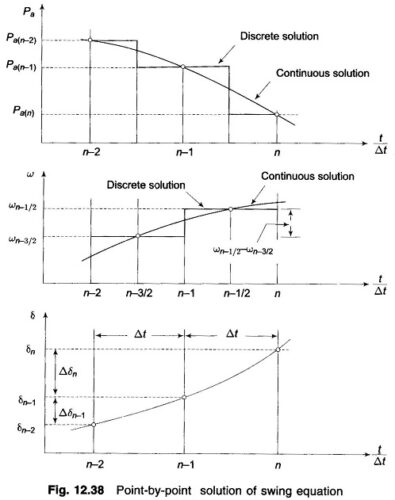Numerical Solution of Swing Equation: Numerical Solution of Swing Equation – In most practical systems, after machine lumping has been done, there are still more than two machines to be considered from the point of view of system stability. Therefore, there is no choice but to solve the swing equation of each machine by a …

## Equal Area Criterion in Power System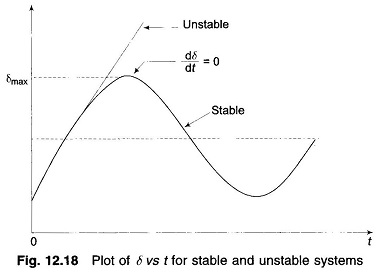Equal Area Criterion in Power System: In a system where one machine is swinging with respect to an infinite bus, it is possible to study transient stability by means of a simple Equal Area Criterion in Power System, without resorting to the numerical solution of a swing equation. Consider the swing equation If the system …

## Transient Stability in Power System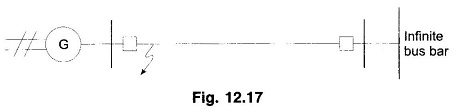Transient Stability in Power System: Transient Stability in Power System – It has been shown already that the dynamics of a single synchronous machine connected to infinite bus bars is governed by the nonlinear differential equation where As said earlier, this equation is known as the swing equation. No closed format solution exists for swing …

## Steady State Stability in Power System

Steady State Stability in Power System: The steady state stability limit of a particular circuit of a power system is defined as the maximum power that can be transmitted to the receiving end without loss of synchronism. Consider the simple system of Fig. 12.14 whose dynamics is described by equations and For determination of Steady …

## Single Machine Infinite Bus in Power System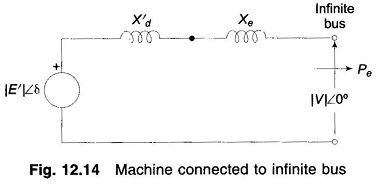Single Machine Infinite Bus in Power System: Figure 12.14 is the circuit model of a Single Machine Infinite Bus in Power System through a line of reactance Xe. In this simple case From Eq. (12.29b) The dynamics of this system are described in Eq. (12.11) as Two Machine System: The case of two finite machines …

## Node Elimination Technique in Power System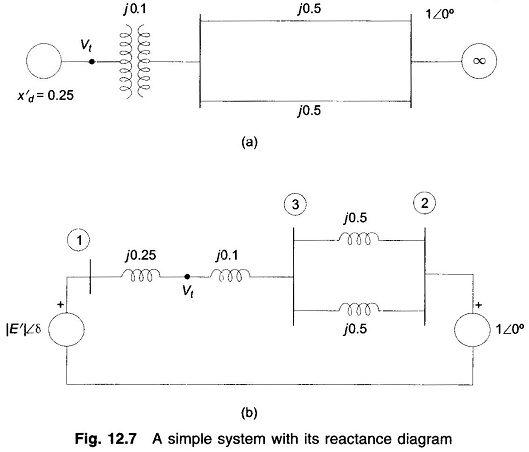Node Elimination Technique in Power System: In stability studies, it has been indicated that the buses to be considered are those which are excited by the internal machine voltages (transient emf’s) and not the load buses which are excited by the terminal voltages of the generators. Therefore, in YBUS formulation for the stability study, the …

## Power Angle Equation of Synchronous Machine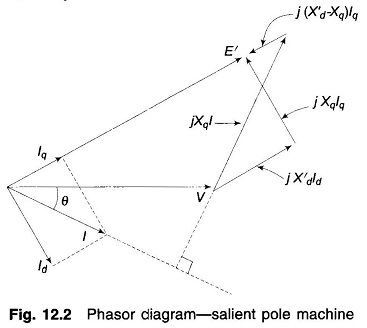Power Angle Equation of Synchronous Machine: Power Angle Equation of Synchronous Machine – In solving the swing equation (Eq. (12.10)), certain simplifying assumptions are usually made. These are: Mechanical power input to the machine (Pm) remains constant during the period of electromechanical transient of interest. In other words, it means that the effect of the …

Scroll to Top